# ISEE Upper Level Quantitative : Data Analysis

## Example Questions

### Example Question #51 : How To Find The Answer From A Table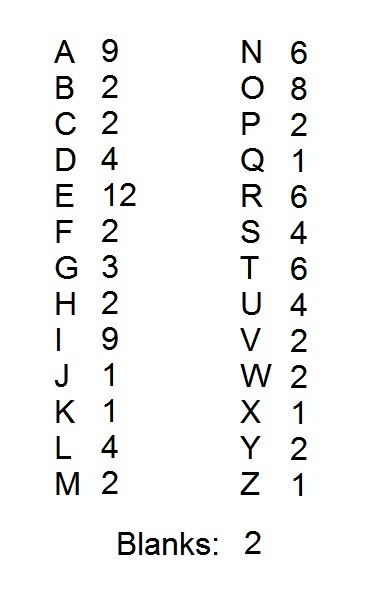A popular word game uses one hundred tiles, each of which is marked with a letter or a blank. The distribution of the tiles is shown above, with each letter paired with the number of tiles marked with that letter. Notice that there are two blank tiles.

All of the tiles are put in a box except for the unique tiles and the blanks. A tile is drawn at random. Which is the greater quantity?

(a) The probability that the tile is a vowel

(b)(Note: "Y" is considered a consonant here)

(b) is the greater quantity

(a) and (b) are equal

It is impossible to determine which is greater from the information given

(a) is the greater quantity

(b) is the greater quantity

Explanation:

There are five unique tiles - "J", "K", "Q", "X", and "Z" - and two blanks, for a total of 7 tiles not placed in the box. Therefore, the box contains 93 tiles.

The number of tiles with each vowel is as follows:

A: 9 tiles

E: 12 tiles

I: 9 tiles

O: 8 tiles

U: 4 tiles

This is a total of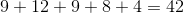vowel tiles. Therefore,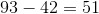consonants remain. Since vowel tiles comprise fewer than half of the remaining tiles, the probability that the draw will be that of a vowel is less than.

### Example Question #551 : Isee Upper Level (Grades 9 12) Quantitative ReasoningA popular word game uses one hundred tiles, each of which is marked with a letter or a blank. The distribution of the tiles is shown above, with each letter paired with the number of tiles marked with that letter. Notice that there are two blank tiles.

All of the tiles are put in a box except for the unique tiles; they are replaced by five tiles from another set - one of each of the five vowels ("Y" is considered a consonant here). A tile is drawn at random. Which is the greater quantity?

(a) The probability that the tile is a consonant

(b)(a) and (b) are equal

(b) is the greater quantity

It is impossible to determine which is greater from the information given

(a) is the greater quantity

(a) is the greater quantity

Explanation:

It is easier to count the tiles that are vowels or blanks than it is to count the tiles marked with consonants. Keeping in mind that one of each vowel has been added, the number of each vowel is as follows:

A: 10 tiles

E: 13 tiles

I: 10 tiles

O: 9 tiles

U: 5 tiles

Blanks: 2 tiles

Add these: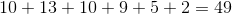tiles not marked with consonants.

Since the five unique tiles - "J", "K", "Q", "X", and "Z" - were replaced by five vowel tiles, the number of tiles remained constant at 100. Therefore, there are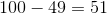consonant tiles; consonants therefore make up more than one half of the tiles, and the probability that a randomly drawn tile will be a consonant is greater than.

### Example Question #73 : Data Analysis And ProbabilityA popular word game uses 100 tiles, each of which is marked with a letter or a blank. The distribution of the tiles is shown above, with each letter paired with the number of tiles marked with that letter. Notice that there are two blank tiles.

All of the tiles are put in a box except for the unique tiles and the blanks. They are replaced by enough randomly selected tiles from a second set to bring the total of tiles back up to 100. Which is the greater quantity?

(a) The number of tiles in the box that are marked with consonants

(b) 50

(b) is the greater quantity

(a) is the greater quantity

It is impossible to determine which is greater from the information given

(a) and (b) are equal

(a) is the greater quantity

Explanation:

The number of tiles with each vowel is as follows:

A: 9 tiles

E: 12 tiles

I: 9 tiles

O: 8 tiles

U: 4 tiles

This is a total ofvowel tiles in the complete set. Considering the two blanks, there are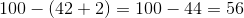consonant tiles.

There are five unique tiles - "J", "K", "Q", "X", and "Z". Removing them leaves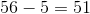consonant tiles. Since the removal of seven tiles and their replacement with seven others leaves the number of tiles in all at 100, at the very least, there are 51 consonant tiles in the new set - more than half.

### Example Question #74 : Data Analysis And Probability

The beverage menu from a coffeehouse reads as follows: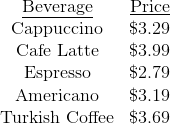Eight friends go out for drinks. Three order cappuccino, two order espresso, one orders Americano, one orders Turkish coffee, and one orders cafe latte. One of the eight agrees to leave the entire amount of the tip, which is to be 20% of the check. How much will be the amount of the tip (round to the nearest dime)?Explanation:

The costs of the beverages will be as follows: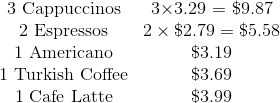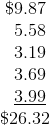20% of this is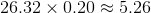, which rounds to \$5.30.

### Example Question #1 : How To Find Median

The median of seven consecutive integers is 129. What is the least integer?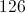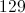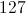It cannot be determined from the information given.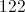Explanation:

The median of seven (an odd number) integers is the one in the middle when the numbers are arranged in ascending order; in this case, it is the fourth lowest. Since the seven integers are consecutive, the lowest integer is three less than the median, or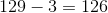.

### Example Question #76 : Data Analysis And Probability

Consider the data set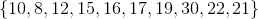Which is the greater quantity?

(a) The mean of this data set

(b) The median of this data set

(a) is greater

It is impossible to tell from the information given

(a) and (b) are equal

(b) is greater

(a) is greater

Explanation:

(a) The mean of this data set is the sum divided by 10: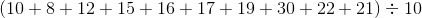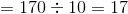(b) The median of a data set with ten elements is the arithmetic mean of the fifth-highest and sixth-highest elements:

The set, arranged, is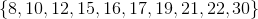The median is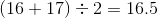This makes (a) the greater quantity

### Example Question #77 : Data Analysis And Probability

Which is the greater quantity?

(a) The median of the data set(b) The median of the data set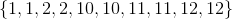(a) is greater.

(b) is greater.

It is impossible to tell from the information given.

(a) and (b) are equal.

(a) and (b) are equal.

Explanation:

Each data set has ten elements, so the median in each case is the arithmetic mean of the fifth-highest and sixth-highest elements. In each data set, these elements are 10 and 10, so the median of each set is 10. Therefore, both quantities are equal.

### Example Question #1 : Median

Consider the data set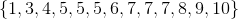.

Which is the greater quantity?

(a) The mean of this set

(b) The median of this set

(a) and (b) are equal.

(a) is greater.

(b) is greater.

It is impossible to tell from the information given.

(b) is greater.

Explanation:

(a) The mean of this set is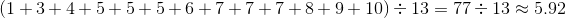.

(b) Since there areelements, the median of this set is the seventh-highest number, which is.

Therefore (b) is the greater quantity.

### Example Question #1 : How To Find Median

Which is the greater quantity?

(a) The mean of the first ten prime numbers

(b) The median of the first ten prime numbers

It is impossible to tell from the information given.

(b) is greater.

(a) is greater.

(a) and (b) are equal.

(a) is greater.

Explanation:

The first ten primes form the data set: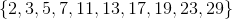(a) Add these primes, and divide by: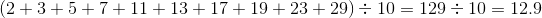(b) The median of a data set with ten elements is the arithmetic mean of the fifth-highest and sixth-highest elements. These areand, so the median is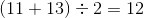.

(a) is the greater quantity.

### Example Question #1 : How To Find Median

A data set with nine elements has mean 10 and median 10. A new data set is formed with these nine elements, plus two new elements, 2 and 18.

Which is the greater quantity?

(a) The mean of the new data set

(b) The median of the new data set

(a) is greater.

It is impossible to tell from the information given.

(b) is greater.

(a) and (b) are equal.

(a) and (b) are equal.

Explanation:

(a) The mean of nine elements is, so, if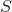is their sum,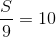and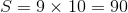. The sum of the new data set is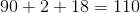. Since the new set haselements, its mean is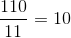.

(b) The median of the nine elements is, so, when they are ranked, the fifth-highest element is. Sinceis less thanandis greater than, when they are added to the set,is the sixth-highest of eleven elements, which is the median.

Therefore, both are equal to.

### All ISEE Upper Level Quantitative Resources